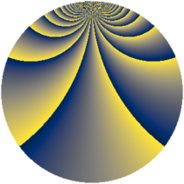# Properties

 Label 1296.2.bhLevel $1296$ Weight $2$ Character orbit 1296.bh Rep. character $\chi_{1296}(37,\cdot)$ Character field $\Q(\zeta_{36})$ Dimension $840$ Sturm bound $432$

# Related objects

## Defining parameters

 Level: $$N$$ $$=$$ $$1296 = 2^{4} \cdot 3^{4}$$ Weight: $$k$$ $$=$$ $$2$$ Character orbit: $$[\chi]$$ $$=$$ 1296.bh (of order $$36$$ and degree $$12$$) Character conductor: $$\operatorname{cond}(\chi)$$ $$=$$ $$432$$ Character field: $$\Q(\zeta_{36})$$ Sturm bound: $$432$$

## Dimensions

The following table gives the dimensions of various subspaces of $$M_{2}(1296, [\chi])$$.

Total New Old
Modular forms 2664 888 1776
Cusp forms 2520 840 1680
Eisenstein series 144 48 96

## Trace form

 $$840 q + 12 q^{2} - 12 q^{4} + 12 q^{5} + 6 q^{8} + O(q^{10})$$ $$840 q + 12 q^{2} - 12 q^{4} + 12 q^{5} + 6 q^{8} - 6 q^{10} + 12 q^{11} - 12 q^{13} + 12 q^{14} - 12 q^{16} + 12 q^{17} - 6 q^{19} + 54 q^{20} - 12 q^{22} + 24 q^{26} - 24 q^{28} + 12 q^{29} - 24 q^{31} + 12 q^{32} + 6 q^{35} - 6 q^{37} + 12 q^{38} - 12 q^{40} - 12 q^{43} + 6 q^{44} - 6 q^{46} + 24 q^{47} - 24 q^{49} + 168 q^{50} - 12 q^{52} + 24 q^{53} + 54 q^{56} + 42 q^{58} + 48 q^{59} - 12 q^{61} - 60 q^{62} - 6 q^{64} + 24 q^{65} - 12 q^{67} + 66 q^{68} - 54 q^{70} + 96 q^{74} - 12 q^{76} + 12 q^{77} - 24 q^{79} + 204 q^{80} - 24 q^{82} - 48 q^{83} + 18 q^{85} + 72 q^{86} - 12 q^{88} - 6 q^{91} - 102 q^{92} - 12 q^{94} + 24 q^{95} - 24 q^{97} + 6 q^{98} + O(q^{100})$$

## Decomposition of $$S_{2}^{\mathrm{new}}(1296, [\chi])$$ into newform subspaces

The newforms in this space have not yet been added to the LMFDB.

## Decomposition of $$S_{2}^{\mathrm{old}}(1296, [\chi])$$ into lower level spaces

$$S_{2}^{\mathrm{old}}(1296, [\chi]) \cong$$ $$S_{2}^{\mathrm{new}}(432, [\chi])$$$$^{\oplus 2}$$